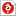# [연세대학교 확률과랜덤변수] 전기전자공학부 전공선택 교과목 확률과랜덤변수 프로젝트 최종 보고서 (전체 MATLAB 코드 및 설명 포함)

최초 등록일
2019.05.23
최종 저작일
2018.06
87페이지/한컴오피스
가격
판매자star*******
다운로드
장바구니

## 소개글

연세대학교 전기전자공학부 확률과랜덤변수 강의의 학기말 프로젝트 보고서입니다.

100점 만점에 99점을 받았고, 이는 전체 조들 가운데 2등에 해당하는 성적입니다.

보고서 내에 문항별 MATLAB 코드와 해설이 모두 포함되어 있습니다.

감사합니다.

## 목차

① Introduction
② Problems and Solutions
③ Discussion
④ Conclusion

## 본문내용

① Introduction

The first question is to learn how the theorems of random variables and PDFs are applied to the real life, especially a Laplacian PDF to measure the speech power.
The second question is about quantizing the signal. We will use several type of quantizer. To calculate boundary point and probabilities, we will use conditional pdf that we had learned Lecture 7.
The third question is about random variable estimation. First, we train the program to acknowledge which one is spam mail and test it, which we call Naive Bayes classifier. We apply MAP theorem which is in Lecture 10.
The fourth question is about using Monte Carlo integration, it is useful when the integration is hard to calculate analytically. We had learned it in Lecture 9, 10.

② Problems and Solutions

※ Question 1 : Practical Engineering Problems

A. Setting Clipping Levels for Speech Signals
To determine the highest amplitude of the speech signal that can be expected to occur a common model is to use a Laplacian PDF for the amplitudes .

없음

## 자료문의

ㆍ이 자료에 대해 궁금한 점을 판매자에게 직접 문의 하실 수 있습니다.
ㆍ상업성 광고글, 욕설, 비방글, 내용 없는 글 등은 운영 방침에 따라 예고 없이 삭제될 수 있습니다.
ㆍ다운로드가 되지 않는 등 서비스 불편사항은 고객센터 1:1 문의하기를 이용해주세요.

## 판매자 정보star*******
회원 소개글이 없습니다.
판매지수
ㆍ판매 자료수
20
ㆍ전체 판매량
11
ㆍ최근 3개월 판매량
3
ㆍ자료후기 점수
받은후기없음
ㆍ자료문의 응답률
받은문의없음
판매자 정보
ㆍ학교정보
• 비공개
ㆍ직장정보
• 비공개
ㆍ자격증
• 비공개
• 위 정보 및 게시물 내용의 진실성에 대하여 해피캠퍼스는 보증하지 아니하며, 해당 정보 및 게시물 저작권과 기타 법적 책임은 자료 등록자에게 있습니다.
위 정보 및 게시물 내용의 불법적 이용, 무단 전재·배포는 금지되어 있습니다.
저작권침해, 명예훼손 등 분쟁요소 발견시 고객센터의 저작권침해 신고센터를 이용해 주시기 바랍니다.최근 본 자료더보기